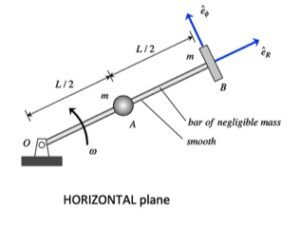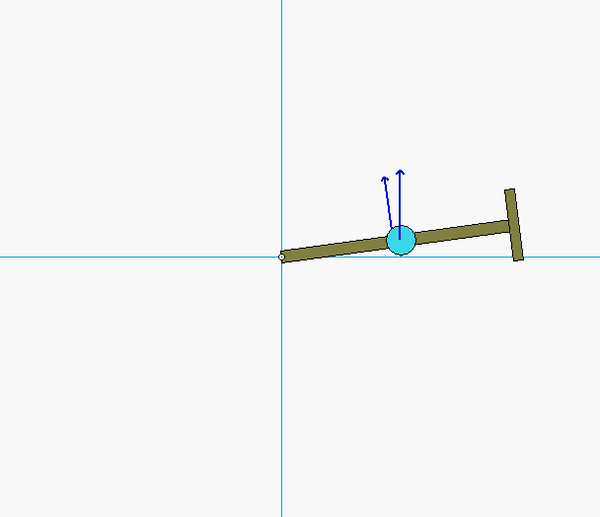# Homework H.4.SYou are asked to investigate the dynamics of this system during the time from releases through to the time of immediately after A strikes B.

• Divide this time of investigation into two segments: 1 to 2, and 2 to 3.
• From times 1 to 2, A slides outward on the arm until it almost impacts B. During this time, angular momentum of A+B+arm  is conserved, as well an energy for that same system is conserved.
• From times 2 to 3, A impacts B. During this time, angular momentum of A+B+arm is conserved; however, due to the impact, energy is NOT conserved. Instead, we will use the coefficient of restitution as the second equation.

HINTS:

STEP 1 - FBD: Draw a SINGLE free body diagram (FBD) of the system of A+P+arm.
STEP 2 - Kinetics: The only force that you will see acting on this system during the full range of time from 1 to 3 is the support force at O. Because this forces acting through point O, angular momentum  about O is conserved throughout. During the pre-impact time of 1 to 2, also write down a conservation of energy equation. During the impact time of 2 to 3, write down the COR equation involving only the radial components of velocity.
STEP 3 - Kinematics: At Instant 1, A and B have only eθ components of velocity. For Instants 2 and 3, A has both eand eθ components of velocity, and B has only eθ components of velocity.
STEP 4 - Solve.

## 14 thoughts on “Homework H.4.S”

1.Matthew Torrisi says:

Do we assume the system is at rest initially? ie. Does B have an initial velocity?

1.Edward James Bregi says:

I believe that the initial angular velocity shows that particle B does have an initial velocity. We can use v = w1L to find the velocity in the etheta direction

1.Matthew Torrisi says:

Thank you!

2.Paul V Richards says:

How do we find A's initial velocity in the e hat R direction? I was able to relate its inital and final velocities in this dirction using the coefficient of restitution, but am stuck on how to move from there to finding that velocity in terms of m, L, w1, and e.

1.hu665 says:

The system is initially at rest, so R dot should be zero

3.Aaron Michael Harp says:

I'm a little confused how to find the e_r portion of the velocity. Assuming A is initally at rest and since B doesn't have an e_r portion of velocity, wouldn't the denominator of the coefficient of restitution equation be 0?

1.CMK says:

AARON: I have recently added some discussion points to the post. Please see the comments there of breaking the problem into two segments of time: pre-impact and during impact.

From this discussion, please note that the coefficient of restitution equation is valid only during the second time segment (during impact).

1.ecasetti says:

Does that mean we have our initial position directly before impact and our final position as directly after impact?

1.CMK says:

Time 1 is on release. Time 2 is immediately before impact. Time 3 is immediately after impact.

You initial time is "1".

From 1 to 2, you have both conservation of angular momentum and conservation of energy. From 2 to 3, you have conservation of angular momentum and the COR equation.

Let me know if this does not help.

4.Sarah Nicole Lavelle says:

When doing the calculations for the step of 2-3 should we assume A is in contact with B so that the distance from O for A is L ? or will distance of A not matter for this step?

1.KT says:

Both are true, we can assume the distance is L, but you don’t really need to use this distance if you use the coefficient of restitution relationship to solve for the final velocity.

2.CMK says:

Yes, A will be at a distance of L from O for both 2 and 3.

5.Darren Lie says:

Does the angular velocity of the bar remain constant as particle A moves outwards and after the impact with B?

1.CMK says:

No, omega is not constant. Angular momentum is a constant, however.